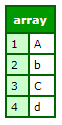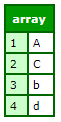# ArraySort

### Description

Sorts array elements numerically or alphanumerically.

### Returns

True, if sort is successful; False, otherwise.

The member function returns the sorted array.

### Function syntax

`ArraySort(array, sortType [, sortOrder, localeSensitive ])`

Or:

`ArraySort(array, callback)`

### History

ColdFusion (2021 release): Made the following changes:

• Sort Member functions accept compare/compareNocase as callback.

ColdFusion (2018 release): Made the following changes:

• Member function returns the sorted array.
• Introduced named parameters.

ColdFusion 10:

ColdFusion MX:

• Changed thrown exceptions: This function can throw the ArraySortSimpleValueException error and ValueNotNumeric error.
• Changed the order in which sorted elements are returned: In a  textnocase , descending sort, this function might return elements in a different sort order than in earlier releases. If sort_type = " textnocase " and sort_order = "desc", ColdFusion processes elements that differ only in case differently from earlier releases, as follows:
• ColdFusion reverses the elements' original order.
• Releases earlier than ColdFusion MX do not change the elements' original order.
For example, in a textnocase, desc sort of d,a,a,b,A, the following occurs:
• ColdFusion MX and later returns d,b,A,a,a
• Releases earlier than ColdFusion MX return d,b,a,a,A

### Parameters

Parameter

Description

array

Name of an array

localeSensitive

Specify if you wish to do a locale sensitive sorting. The default value is false.

sortType

• numeric: sorts numbers
• text: sorts text alphabetically, taking case into account (also known as case sensitive). All letters of one case precede the first letter of the other case:
• aabzABZ , if sort_order = " asc " (ascending sort)- ZBAzbaa, if sort_order = "desc" (descending sort)
• textnocase : sorts text alphabetically, without regard to case (also known as case-insensitive). A letter in varying cases precedes the next letter:
• aAaBbBzzZ, in an ascending sort; preserves original intra-letter order - ZzzBbBaAa, in a descending sort; reverses original intra-letter order

sortOrder

• asc - ascending sort order. Default.
• aabzABZ or aAaBbBzzZ, depending on value of sort_type, for letters- from smaller to larger, for numbers
• desc - descending sort order.
• ZBAzbaa or ZzzBbBaAa, depending on value of sort_type, for letters- from larger to smaller, for numbers

callback

A function which take two elements of the array, and returns whether the first is less than (-1), equal to (0) or greater than (1) the second one (similar to how  compare () works for strings).

### Throws

If an array element is something other than a simple element, this function throws an ArraySortSimpleValueException error. If sort_type is numeric and an array element is not numeric, this function throws a ValueNotNumeric error.

### Usage

In ColdFusion 10, added support for all Java supported locale-specific characters (including support for umlaut characters). A flag for this support has been added for sorttype = "text" or sorttype = "textnocase".

### Example

```<!--- This example shows ArraySort. --->
<cfquery name = "GetEmployeeNames" datasource = "cfdocexamples">
SELECT FirstName, LastName FROM Employees
</cfquery>
<!--- Create an array. --->
<cfset myArray = ArrayNew(1)>
<!--- Loop through the query and append these names successively to the last element. --->
<cfloop query = "GetEmployeeNames">
<cfset temp = ArrayAppend(myArray, "#FirstName# #LastName#")>
</cfloop>
<!--- Show the resulting array as a list. --->
<cfset myList = ArrayToList(myArray, ",")>
<!--- Sort that array in descending order alphabetically. --->
<cfset isSuccessful = ArraySort(myArray, "textnocase", "desc")>
...```
```<cfscript>
authors = [
{firstName="Witi", lastName="Ihimaera"},
{firstName="Patricia", lastName="Grace"},
{firstName="Alan", lastName="Duff"},
{firstName="Lee", lastName="Tamahori"}, // OK: not an author
{firstName="Keri", lastName="Hulme"}
];
arraySort(
authors,
function (e1, e2){
return compare(e1.lastName, e2.lastName);
}
);
writeDump(authors);
</cfscript>```

Note that the callback function does not need to be inline, as in the example; it can be any predefined user-defined function.

OutputExample using compareNoCase as callback.

```<cfscript>
arrayToSort = ["d","C","b","A"];
sortedArray = arrayToSort.sort(compareNoCase);
writeDump(sortedArray)
</cfscript>```

OutputExample using compare as callback.

```<cfscript>
arrayToSort = ["d","C","b","A"];
sortedArray = arrayToSort.sort(compareNoCase);
writeDump(sortedArray)
</cfscript>```

Output